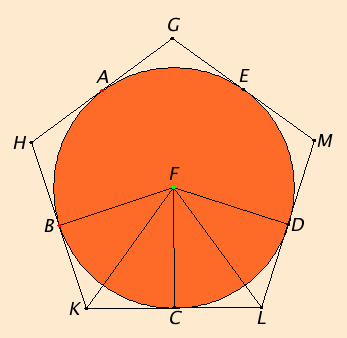# Proposition 12

To circumscribe an equilateral and equiangular pentagon about a given circle.

Let ABCDE be the given circle.

It is required to circumscribe an equilateral and equiangular pentagon about the circle ABCDE.

IV.11

Let A, B, C, D, and E be conceived to be the angular points of the inscribed pentagon, so that the circumferences AB, BC, CD, DE, and EA are equal.

Draw GH, HK, KL, LM, and MG through A, B, C, D, and E touching the circle. Take the center F of the circle ABCDE, and join FB, FK, FC, FL, and FD.III.18

Then, since the straight line KL touches the circle ABCDE at C, and FC has been joined from the center F to the point of contact at C, therefore FC is perpendicular to KL. Therefore each of the angles at C is right.

For the same reason the angles at the points B and D are also right.

I.47

And, since the angle FCK is right, therefore the square on FK equals the sum of the squares on FC and CK.

I.47

For the same reason the square on FK also equals the sum of the squares on FB and BK, so that the sum of the squares on FC and CK equals the sum of the squares on FB and BK, of which the square on FC equals the square on FB, therefore the remaining square on CK equals the square on BK.

Therefore BK equals CK.

I.8

And, since FB equals FC, and FK is common, the two sides BF and FK equal the two sides CF and FK, and the base BK equals the base CK, therefore the angle BFK equals the angle KFC, and the angle BKF equals the angle FKC. Therefore the angle BFC is double the angle KFC, and the angle BKC double the angle FKC.

For the same reason the angle CFD is also double the angle CFL, and the angle DLC double the angle FLC.

III.27

Now, since the circumference BC equals CD, the angle BFC also equals the angle CFD.

And the angle BFC is double the angle KFC, and the angle DFC double the angle LFC, therefore the angle KFC also equals the angle LFC.

I.26

But the angle FCK also equals the angle FCL, therefore FKC and FLC are two triangles having two angles equal to two angles and one side equal to one side, namely FC which is common to them, therefore they will also have the remaining sides equal to the remaining sides, and the remaining angle to the remaining angle, therefore the straight line KC equals CL, and the angle FKC equals the angle FLC.

And, since KC equals CL, therefore KL is double KC.

For the same reason it can be proved that HK is also double BK.

And BK equals KC, therefore HK also equals KL.

Similarly each of the straight lines HG, GM, and ML can also be proved equal to each of the straight lines HK and KL, therefore the pentagon GHKLM is equilateral.

I say next that it is also equiangular.

For, since the angle FKC equals the angle FLC, and the angle HKL was proved double the angle FKC, and the angle KLM double the angle FLC, therefore the angle HKL also equals the angle KLM.

Similarly each of the angles KHG, HGM, and GML can also be proved equal to each of the angles HKL and KLM. Therefore the five angles GHK, HKL, KLM, LMG, and MGH equal one another.

Therefore the pentagon GHKLM is equiangular.

And it was also proved equilateral, and it has been circumscribed about the circle ABCDE.

Q.E.F.

## Guide

This construction depends on the last. First, inscribe a regular pentagon in the circle, then take tangents to the circle at the five vertices of the inscribed pentagon. Euclid didn’t actually give the construction for drawing tangents, but the Corollary to Proposition III.16 indicates how it should be constructed. The result will be a circumscribed pentagon. This method generally works to create a regular circumscribed n-gon given a regular inscribed n-gon.

Conversely, if you have a regular circumscribed n-gon, then you can connect the points of tangency in sequence to get a regular inscribed n-gon.